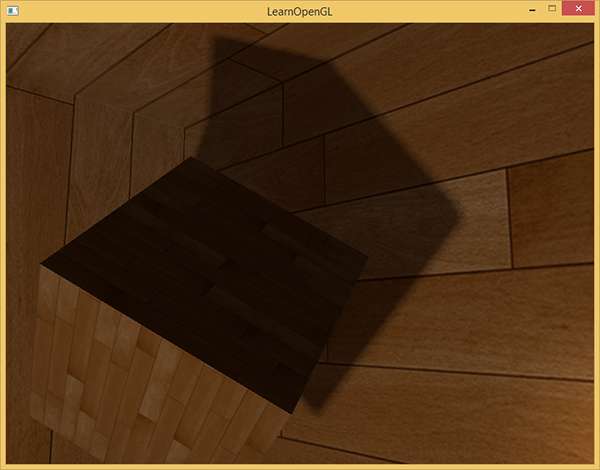# 点光源阴影

Note

Important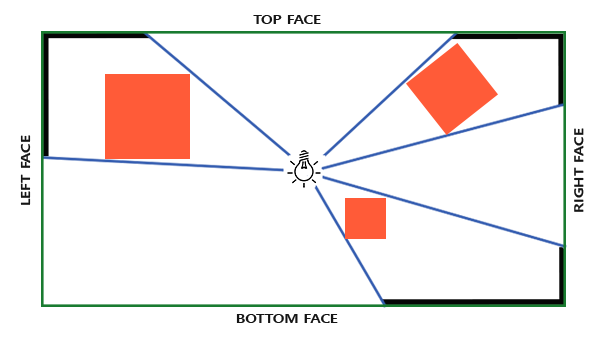## 生成深度立方体贴图

for(int i = 0; i < 6; i++)
{
GLuint face = GL_TEXTURE_CUBE_MAP_POSITIVE_X + i;
glFramebufferTexture2D(GL_FRAMEBUFFER, GL_DEPTH_ATTACHMENT, face, depthCubemap, 0);
BindViewMatrix(lightViewMatrices[i]);
RenderScene();
}


GLuint depthCubemap;
glGenTextures(1, &depthCubemap);


const GLuint SHADOW_WIDTH = 1024, SHADOW_HEIGHT = 1024;
glBindTexture(GL_TEXTURE_CUBE_MAP, depthCubemap);
for (GLuint i = 0; i < 6; ++i)
glTexImage2D(GL_TEXTURE_CUBE_MAP_POSITIVE_X + i, 0, GL_DEPTH_COMPONENT,


glTexParameteri(GL_TEXTURE_CUBE_MAP, GL_TEXTURE_MAG_FILTER, GL_NEAREST);
glTexParameteri(GL_TEXTURE_CUBE_MAP, GL_TEXTURE_MIN_FILTER, GL_NEAREST);
glTexParameteri(GL_TEXTURE_CUBE_MAP, GL_TEXTURE_WRAP_S, GL_CLAMP_TO_EDGE);
glTexParameteri(GL_TEXTURE_CUBE_MAP, GL_TEXTURE_WRAP_T, GL_CLAMP_TO_EDGE);
glTexParameteri(GL_TEXTURE_CUBE_MAP, GL_TEXTURE_WRAP_R, GL_CLAMP_TO_EDGE);


glBindFramebuffer(GL_FRAMEBUFFER, depthMapFBO);
glFramebufferTexture(GL_FRAMEBUFFER, GL_DEPTH_ATTACHMENT, depthCubemap, 0);
glDrawBuffer(GL_NONE);
glBindFramebuffer(GL_FRAMEBUFFER, 0);


// 1. first render to depth cubemap
glBindFramebuffer(GL_FRAMEBUFFER, depthMapFBO);
glClear(GL_DEPTH_BUFFER_BIT);
RenderScene();
glBindFramebuffer(GL_FRAMEBUFFER, 0);
// 2. then render scene as normal with shadow mapping (using depth cubemap)
glViewport(0, 0, SCR_WIDTH, SCR_HEIGHT);
glClear(GL_COLOR_BUFFER_BIT | GL_DEPTH_BUFFER_BIT);
glBindTexture(GL_TEXTURE_CUBE_MAP, depthCubemap);
RenderScene();


### 光空间的变换

GLfloat aspect = (GLfloat)SHADOW_WIDTH/(GLfloat)SHADOW_HEIGHT;
GLfloat near = 1.0f;
GLfloat far = 25.0f;


std::vector<glm::mat4> shadowTransforms;
glm::lookAt(lightPos, lightPos + glm::vec3(1.0,0.0,0.0), glm::vec3(0.0,-1.0,0.0));
glm::lookAt(lightPos, lightPos + glm::vec3(-1.0,0.0,0.0), glm::vec3(0.0,-1.0,0.0));
glm::lookAt(lightPos, lightPos + glm::vec3(0.0,1.0,0.0), glm::vec3(0.0,0.0,1.0));
glm::lookAt(lightPos, lightPos + glm::vec3(0.0,-1.0,0.0), glm::vec3(0.0,0.0,-1.0));
glm::lookAt(lightPos, lightPos + glm::vec3(0.0,0.0,1.0), glm::vec3(0.0,-1.0,0.0));
glm::lookAt(lightPos, lightPos + glm::vec3(0.0,0.0,-1.0), glm::vec3(0.0,-1.0,0.0));


### 深度着色器

#version 330 core
layout (location = 0) in vec3 position;

uniform mat4 model;

void main()
{
gl_Position = model * vec4(position, 1.0);
}


#version 330 core
layout (triangles) in;
layout (triangle_strip, max_vertices=18) out;

out vec4 FragPos; // FragPos from GS (output per emitvertex)

void main()
{
for(int face = 0; face < 6; ++face)
{
gl_Layer = face; // built-in variable that specifies to which face we render.
for(int i = 0; i < 3; ++i) // for each triangle's vertices
{
FragPos = gl_in[i].gl_Position;
EmitVertex();
}
EndPrimitive();
}
}


#version 330 core
in vec4 FragPos;

uniform vec3 lightPos;
uniform float far_plane;

void main()
{
// get distance between fragment and light source
float lightDistance = length(FragPos.xyz - lightPos);

// map to [0;1] range by dividing by far_plane
lightDistance = lightDistance / far_plane;

// write this as modified depth
gl_FragDepth = lightDistance;
}


## 万向阴影贴图

glViewport(0, 0, SCR_WIDTH, SCR_HEIGHT);
glClear(GL_COLOR_BUFFER_BIT | GL_DEPTH_BUFFER_BIT);
// ... send uniforms to shader (including light's far_plane value)
glActiveTexture(GL_TEXTURE0);
glBindTexture(GL_TEXTURE_CUBE_MAP, depthCubemap);
// ... bind other textures
RenderScene();


#version 330 core
layout (location = 0) in vec3 position;
layout (location = 1) in vec3 normal;
layout (location = 2) in vec2 texCoords;

out vec2 TexCoords;

out VS_OUT {
vec3 FragPos;
vec3 Normal;
vec2 TexCoords;
} vs_out;

uniform mat4 projection;
uniform mat4 view;
uniform mat4 model;

void main()
{
gl_Position = projection * view * model * vec4(position, 1.0f);
vs_out.FragPos = vec3(model * vec4(position, 1.0));
vs_out.Normal = transpose(inverse(mat3(model))) * normal;
vs_out.TexCoords = texCoords;
}


#version 330 core
out vec4 FragColor;

in VS_OUT {
vec3 FragPos;
vec3 Normal;
vec2 TexCoords;
} fs_in;

uniform sampler2D diffuseTexture;
uniform samplerCube depthMap;

uniform vec3 lightPos;
uniform vec3 viewPos;

uniform float far_plane;

{
[...]
}

void main()
{
vec3 color = texture(diffuseTexture, fs_in.TexCoords).rgb;
vec3 normal = normalize(fs_in.Normal);
vec3 lightColor = vec3(0.3);
// Ambient
vec3 ambient = 0.3 * color;
// Diffuse
vec3 lightDir = normalize(lightPos - fs_in.FragPos);
float diff = max(dot(lightDir, normal), 0.0);
vec3 diffuse = diff * lightColor;
// Specular
vec3 viewDir = normalize(viewPos - fs_in.FragPos);
vec3 reflectDir = reflect(-lightDir, normal);
float spec = 0.0;
vec3 halfwayDir = normalize(lightDir + viewDir);
spec = pow(max(dot(normal, halfwayDir), 0.0), 64.0);
vec3 specular = spec * lightColor;
vec3 lighting = (ambient + (1.0 - shadow) * (diffuse + specular)) * color;

FragColor = vec4(lighting, 1.0f);
}


float ShadowCalculation(vec3 fragPos)
{
vec3 fragToLight = fragPos - lightPos;
float closestDepth = texture(depthMap, fragToLight).r;
}


closestDepth值现在在0到1的范围内了，所以我们先将其转换回0到far_plane的范围，这需要把他乘以far_plane：

closestDepth *= far_plane;


float currentDepth = length(fragToLight);


float bias = 0.05;
float shadow = currentDepth -  bias > closestDepth ? 1.0 : 0.0;


float ShadowCalculation(vec3 fragPos)
{
// Get vector between fragment position and light position
vec3 fragToLight = fragPos - lightPos;
// Use the light to fragment vector to sample from the depth map
float closestDepth = texture(depthMap, fragToLight).r;
// It is currently in linear range between [0,1]. Re-transform back to original value
closestDepth *= far_plane;
// Now get current linear depth as the length between the fragment and light position
float currentDepth = length(fragToLight);
float bias = 0.05;
float shadow = currentDepth -  bias > closestDepth ? 1.0 : 0.0;

}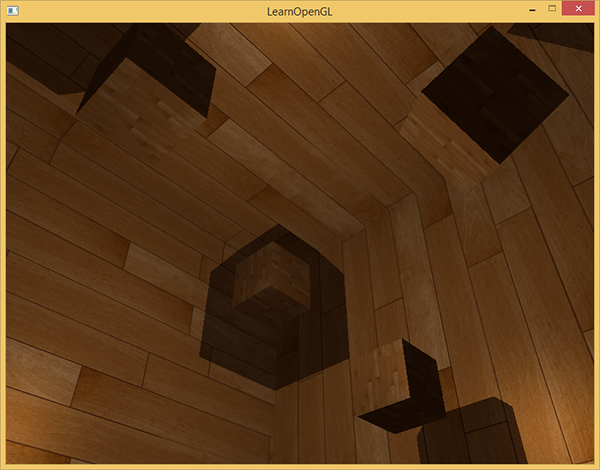### 显示立方体贴图深度缓冲

FragColor = vec4(vec3(closestDepth / far_plane), 1.0);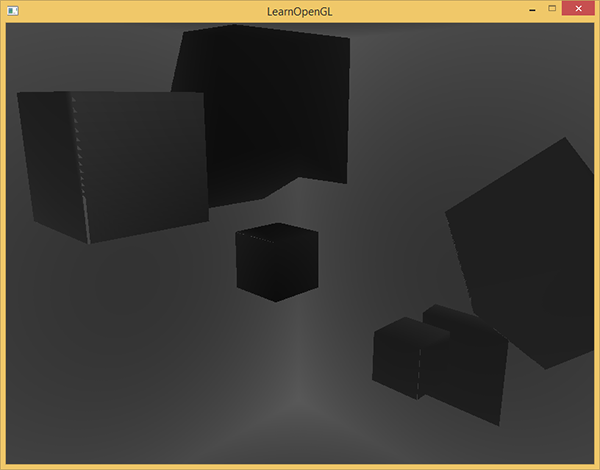## PCF

float shadow = 0.0;
float bias = 0.05;
float samples = 4.0;
float offset = 0.1;
for(float x = -offset; x < offset; x += offset / (samples * 0.5))
{
for(float y = -offset; y < offset; y += offset / (samples * 0.5))
{
for(float z = -offset; z < offset; z += offset / (samples * 0.5))
{
float closestDepth = texture(depthMap, fragToLight + vec3(x, y, z)).r;
closestDepth *= far_plane;   // Undo mapping [0;1]
if(currentDepth - bias > closestDepth)
}
}
}
shadow /= (samples * samples * samples);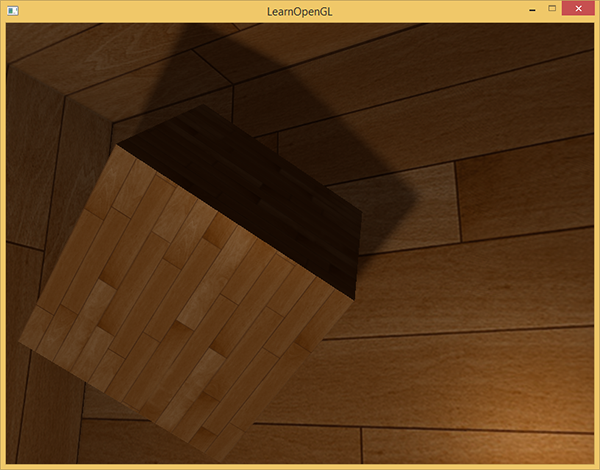vec3 sampleOffsetDirections = vec3[]
(
vec3( 1,  1,  1), vec3( 1, -1,  1), vec3(-1, -1,  1), vec3(-1,  1,  1),
vec3( 1,  1, -1), vec3( 1, -1, -1), vec3(-1, -1, -1), vec3(-1,  1, -1),
vec3( 1,  1,  0), vec3( 1, -1,  0), vec3(-1, -1,  0), vec3(-1,  1,  0),
vec3( 1,  0,  1), vec3(-1,  0,  1), vec3( 1,  0, -1), vec3(-1,  0, -1),
vec3( 0,  1,  1), vec3( 0, -1,  1), vec3( 0, -1, -1), vec3( 0,  1, -1)
);


float shadow = 0.0;
float bias = 0.15;
int samples = 20;
float viewDistance = length(viewPos - fragPos);
for(int i = 0; i < samples; ++i)
{
float closestDepth = texture(depthMap, fragToLight + sampleOffsetDirections[i] * diskRadius).r;
closestDepth *= far_plane;   // Undo mapping [0;1]
if(currentDepth - bias > closestDepth)
}


float diskRadius = (1.0 + (viewDistance / far_plane)) / 25.0;


PCF算法的结果如果没有变得更好，也是非常不错的，这是柔和的阴影效果：